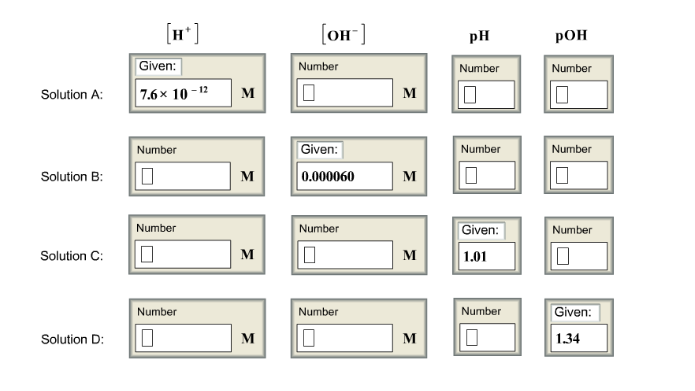Chemistry Practice Problems pH and pOH Practice Problems Solution: Complete this table of values for four aqueous sol...

🤓 Based on our data, we think this question is relevant for Professor Islam's class at UIC.

# Solution: Complete this table of values for four aqueous solutions at 25°c

###### Problem

Complete this table of values for four aqueous solutions at 25°c

###### Solution

pH  of a solution is found by: pH = -log[H+]

pOH of a solution is found by: pOH = -log[OH-]

The relationship between pH and pOH is: pH + pOH = 14

[H+] [OH-] = 1.0 x 10-14

For solution A:

The concentration of [H+ given is 7.6x10-12M

To find pH:

pH = -log[H+]          pH = -log [7.6x10-12]          pH = 11.12View Complete Written SolutionpH and pOH

pH and pOH

#### Q. Calculate the concentrations of H 3O+ and OH− in each of the following solutions:i) Human blood (pH 7.40)ii) A cola beverage (pH 2.8)

Solved • Fri Feb 16 2018 13:07:54 GMT-0500 (EST)

pH and pOH

#### Q. What is the pH of an aqueous solution with the hydronium ion concentration [H  3O+] = 2 x 10 -14 M ?

Solved • Fri Feb 16 2018 12:14:30 GMT-0500 (EST)

pH and pOH

#### Q. Which of these statements are true for a neutral, aqueous solution at 25 degree C? More than one can be correct. a. pH = 7.00b. pOH = 7.00c. [H+] = [O...

Solved • Thu Jan 04 2018 11:01:14 GMT-0500 (EST)

pH and pOH

#### Q. Why is the pH of distilled water usually less than 7?

Solved • Tue Dec 19 2017 11:33:40 GMT-0500 (EST)